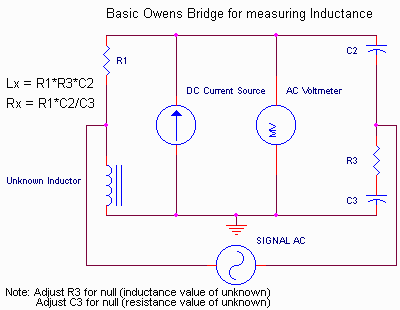An Inductance Bridge you can build

Background / Introduction

Measurement of inductance is sometimes necessary in your amplifier (or whatever) developments. For amplifiers, characterizing an unknown transformer will allow you to determine what kinds of circuits the transformer will likely work with. Inductors can be analyzed to determine their fit for power supply use and/or amplifier use. You also may want to determine how much DC or AC signal the transformer or inductor is likely to handle.

This series of articles describes Owens Bridge inductance "meters" that are relatively easy to build and calibrate. Several different "levels" of instruments will be described, depending on how ambitious you are. The simplest allows you to just measure inductance. More sophisticated versions allow you to measure inductance as a function of DC and AC excitation levels, so that you can determine how much power or DC the inductor or transformer is likely to handle.

The Owens bridge was selected for a basis of these instruments for a few reasons:

1. The adjustment for the inductive and the resistive parts of the unknown inductor are independent.
2. It is easy to "calibrate" the instrument and keep it calibrated. (accurate results).
3. DC and AC can independently applied, and the inductance measurement is not dependent on the frequency.

In this first article, we will describe the Owens Bridge, and outline what kinds of instruments will be described in later articles.

Simplified SchematicDescription

The basic concept behind any bridge type of instrument is a null measurement. You make adjustments of at least one of the values in the bridge, and look for a MINIMUM (null) on the detector. Since a normal inductor has reactive (inductance) components and resistive components, at least two adjustments are necessary. For the Owens bridge, by examining the formulas shown in the schematic above, you will notice that R3 appears only in the inductive part of the "unknown" balance, and C3 appears only in the resistive part. This is very nice, since it means that there is no interaction during the balance. So, we can simplify the adjustments and still get accurate results. I will point out that since a capacitor is varied to obtain the resistance part of the inductor, we will not "calibrate" that portion of the instrument, since an ohmmeter will give you results that are probably more accurate (unless you get into ultrasonic or RF signals where you might want to measure the effects of the AC resistance (in phase component, not inductive or capacitive).

Notice as well that there is a capacitor (C2 or C3) that blocks any potential DC that might be placed across the AC source. This means we can place DC current in the circuit without it affecting the AC source.

Notice further that the formulas for the inductance null (and resistance null) are independent of applied AC frequency. This means that we are also free to explore the effects of frequency on inductance value.

There is one limitation with this bridge. Notice that the DC current is placed directly in parallel with the detector. This means that the current source has to be relatively high impedance, and it must be free of ripple (in a null instrument, you are looking for a minimum level at the detector, so ripple affects the degree of null you can get).

So what kind of instruments can we build?

For the simplest case, I'll show a real bridge circuit that requires only an AC meter to measure inductance at one frequency and one AC level. This will use a bank of switched resistors as part of the bridge. The inductance value can then unambiguously read from the switch positions, making the calibration "easy".

For the next complicated version, we will add capability of changing the DC current flowing through the "unknown" inductor. This can be either an external DC voltage source (with current source conversion shown schematically in the instrument) or an entire DC supply added to the instrument.

Later, we will add capability to change the AC signal level and signal frequency.

-Steve